Question

# Explain the code: Exercise 22: Write a program that creates three random double variables a, b,...

Explain the code:

1. Exercise 22: Write a program that creates three random double variables a, b, and c and assigns them values between 0 and 1 using the Math.random( ) method mentioned in the preceding exercise. It then does all of the following:

It prints out the three values.

It prints "All are tiny" if all three values are less than 0.5.

It prints out "One is tiny" if exactly one of the three values is less than 0.5.

It prints out "Only two are tiny" if exactly two of the three values are less than 0.5.

it prints if “None is tiny” if none of the values are less than 0.5.

```public class ThreeRandom {

public static void main(String[] args) {
// Generate three random numbers
double a = Math.random(), b = Math.random(), c = Math.random();
// print those random numbers
System.out.println("Numbers are " + a + ", " + b + " and " + c);

if (a < 0.5 && b < 0.5 && c < 0.5) {    // If all numbers are less than 0.5
System.out.println("All are tiny"); // print that all are tiny
} else if((a < 0.5 && b < 0.5) || (b < 0.5 && c < 0.5) || (a < 0.5 && c < 0.5)) {   // if two of these numbers are less than 0.5
System.out.println("Only two are tiny");    // print that two of them are tiny
} else if(a < 0.5 || b < 0.5 || c < 0.5) {  // // if only one of these numbers are less than 0.5
System.out.println("One is tiny");  // print that one of them is tiny
} else {    // if none of them are less than 0.5
System.out.println("None is tiny"); // print that none are tiny
}
}
}
```

#### Earn Coins

Coins can be redeemed for fabulous gifts.

Similar Homework Help Questions
• ### Variables lab Write a program that creates three variables: an int, a double, and a String. Put the value 113 into t...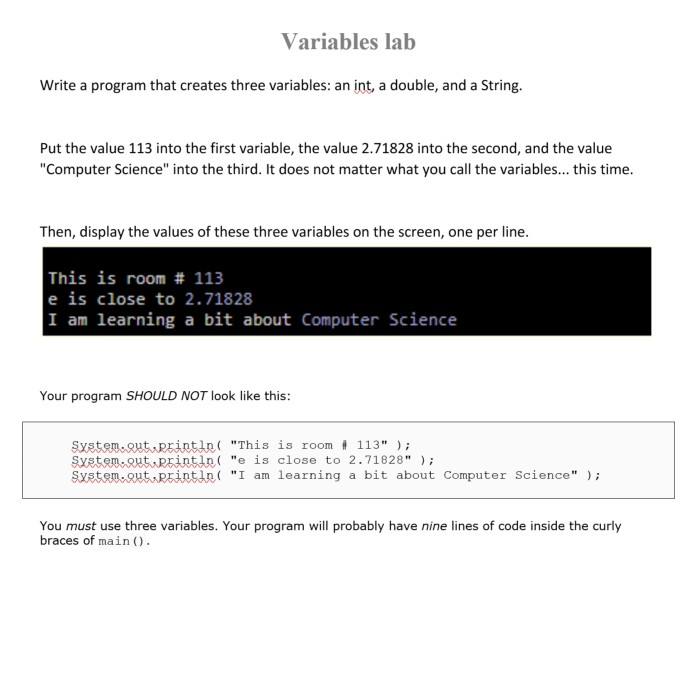Variables lab Write a program that creates three variables: an int, a double, and a String. Put the value 113 into the first variable, the value .71828 into the second, and the value Computer Science" into the third. It does not matter what you call the variables... this time. Then, display the values of these three variables on the screen, one per line. This is room # 113 e is close to 2.71828 I am learning a bit about Computer...

• ### I need a c++ code please. 32. Program. Write a program that creates an integer constant...

I need a c++ code please. 32. Program. Write a program that creates an integer constant called SIZE and initialize to the size of the array you will be creating. Use this constant throughout your functions you will implement for the next parts and your main program. Also, in your main program create an integer array called numbers and initialize it with the following values (Please see demo program below): 68, 100, 43, 58, 76, 72, 46, 55, 92, 94,...

• ### IN JAVA 3 ZIPS, What Order?: Write a program named ZipOrder that the reads in three...

IN JAVA 3 ZIPS, What Order?: Write a program named ZipOrder that the reads in three zip codes from standard input and prints to standard output one of three words: "ASCENDING", "DESCENDING", "UNSORTED". The zip codes are guaranteed to be distinct from each other. In particular: if each zip code read in is greater than to the previous one, "ASCENDING"  is printed. if each zip code read in is less than to the previous one, "DESCENDING" is printed. otherwise, "UNSORTED" is...

• ### See the image below and write the code using C++, without using variables, only recursion. 3....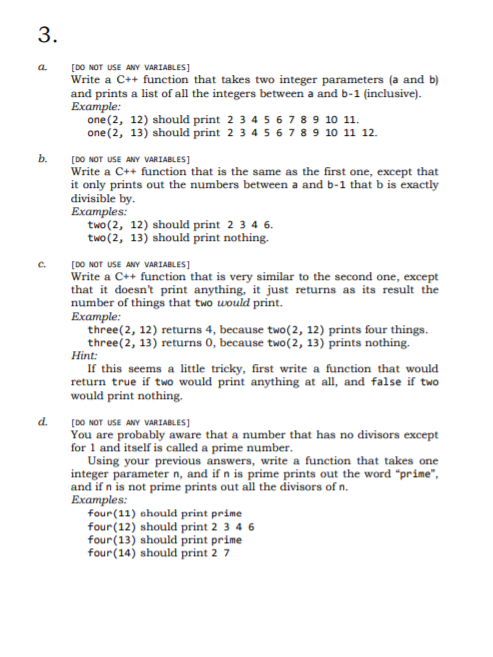See the image below and write the code using C++, without using variables, only recursion. 3. 5 a [DO NOT USE ANY VARIABLES Write a C++ function that takes two integer parameters (a and b) and prints a list of all the integers between a and b-1 (inclusive). one (2, 12) should print 2 34567 8 9 10 11. one (2, 13) should print 2 34567 8 9 10 11 12 b. [D NOT USE ANY VARIABLES Write a C++...

• ### Exercise 2 Using javascript (visual studio code) (a) Write code that creates 5 item objects (records)...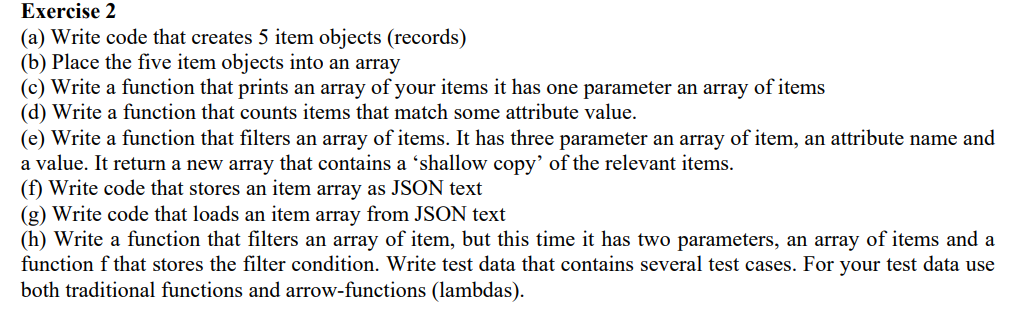Exercise 2 Using javascript (visual studio code) (a) Write code that creates 5 item objects (records) (b) Place the five item objects into an array (c) Write a function that prints an array of your items it has one parameter an array of items (d) Write a function that counts items that match some attribute value. (e) Write a function that filters an array of items. It has three parameter an array of item, an attribute name and a value....

• ### Write a program to pack boxes with blobs. Write two classes and a driver program. A...

Write a program to pack boxes with blobs. Write two classes and a driver program. A Blob class implements the following interface. (Defines the following methods.) public interface BlobInterface { /** getWeight accessor method. @return the weight of the blob as a double */ public double getWeight(); /** toString method @return a String showing the weight of the Blob */ public String toString(); } A Blob must be between 1 and 4 pounds, with fractional weights allowed. A default constructor...

• ### In Java. Write a program in a single file that: Main: Creates 10 random doubles, all...

In Java. Write a program in a single file that: Main: Creates 10 random doubles, all between 1 and 11, Calls a class that writes 10 random doubles to a text file, one number per line. Calls a class that reads the text file and displays all the doubles and their sum accurate to two decimal places. There has to be three classes, main for create random numbers, one to write , one to read all in the same file...

• ### In a file called First SecondNext.java, write a program that: • Asks the user to enter...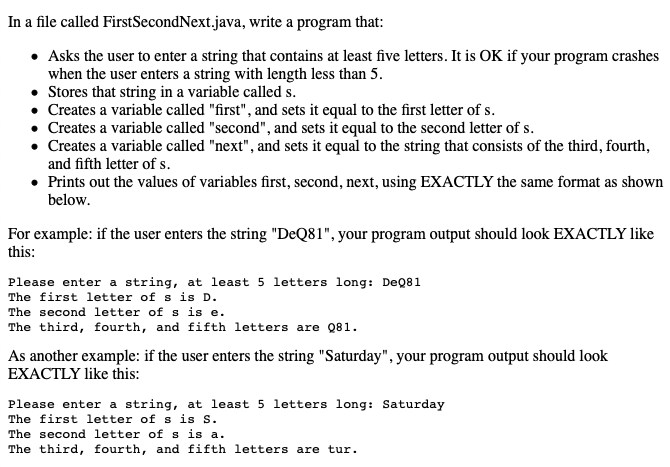In a file called First SecondNext.java, write a program that: • Asks the user to enter a string that contains at least five letters. It is OK if your program crashes when the user enters a string with length less than 5. • Stores that string in a variable called s. • Creates a variable called "first", and sets it equal to the first letter of s. • Creates a variable called "second", and sets it equal to the second...

• ### Writing the code in IDLE. Make sure the code is working. thank you so much Create the following turtle pattern. Using the turtle and random modules, write a program that creates an output as...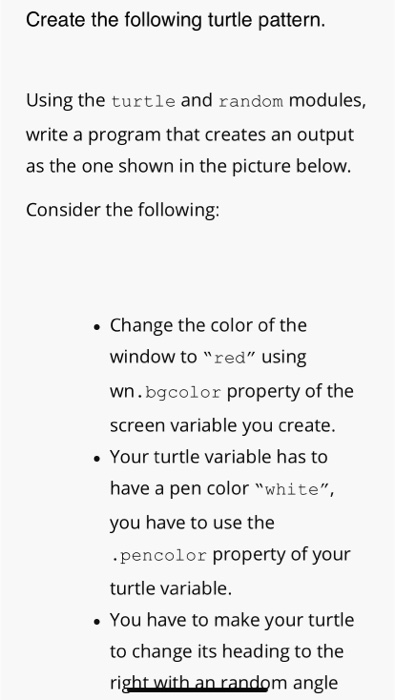Writing the code in IDLE. Make sure the code is working. thank you so much Create the following turtle pattern. Using the turtle and random modules, write a program that creates an output as the one shown in the picture below. Consider the following: . Change the color of the window to "red" using wn.bgcolor property of the screen variable you create. . Your turtle variable has to have a pen color "white", you have to use the .pencolor property...

• ### Part 2 -Arrays We can use the Math.random method to generate random integers. For example, Math.r...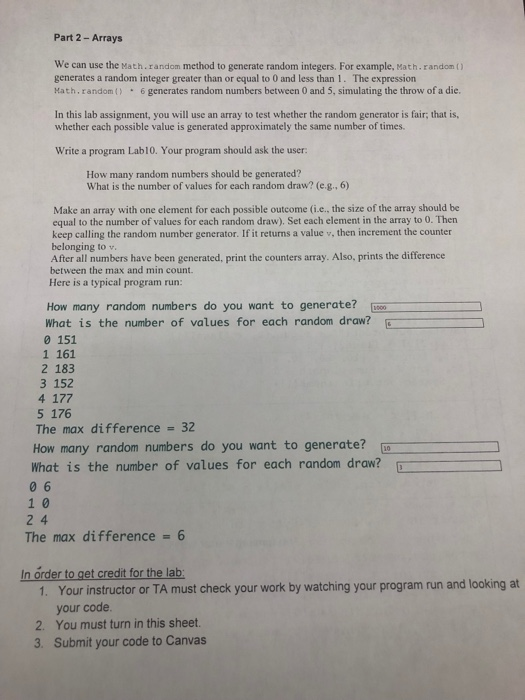Please write a JAVA program according to following requirement. Thanks Part 2 -Arrays We can use the Math.random method to generate random integers. For example, Math.random () generates a random integer greater than or equal to 0 and less than 1. The expression Math. random) 6generates random numbers between 0 and 5, simulating the throw of a die. In this lab assignment, you will use an array to test whether the random generator is fair; that is, whether each possible...